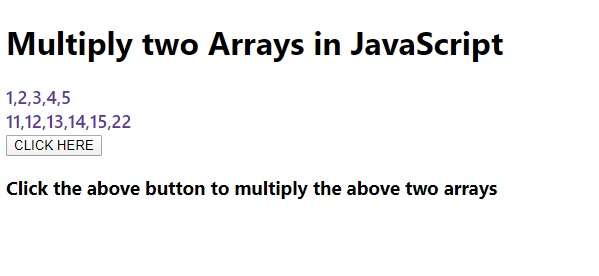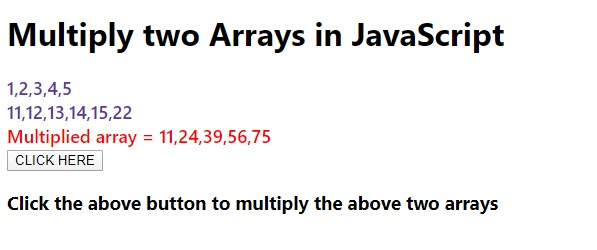# How to multiply two Arrays in JavaScript?

Following is the code to multiply two arrays in JavaScript −

## Example

Live Demo

<!DOCTYPE html>
<html lang="en">
<meta charset="UTF-8" />
<meta name="viewport" content="width=device-width, initial-scale=1.0" />
<title>Document</title>
<style>
body {
font-family: "Segoe UI", Tahoma, Geneva, Verdana, sans-serif;
}
.result,.sample {
font-size: 18px;
font-weight: 500;
color: rebeccapurple;
}
.result {
color: red;
}
</style>
<body>
<h1>Multiply two Arrays in JavaScript</h1>
<div class="sample"></div>
<div class="result"></div>
<h3>Click the above button to multiply the above two arrays</h3>
<script>
let BtnEle = document.querySelector(".Btn");
let resEle = document.querySelector(".result");
let sampleEle = document.querySelector(".sample");
let arr = [1, 2, 3, 4, 5];
let arr1 = [11, 12, 13, 14, 15, 22];
let multArray = [];
sampleEle.innerHTML = arr + "<br>" + arr1 + "<br>";
for (let i = 0; i < Math.min(arr.length, arr1.length); i++) {
multArray[i] = arr[i] * arr1[i];
}
resEle.innerHTML = "Multiplied array = " + multArray;
});
</script>
</body>
</html>

## Output

The above code will produce the following output −